Homework Help Question & Answers

# 4. Proving constant returns to scale A production function expresses the relationship between inputs, such as...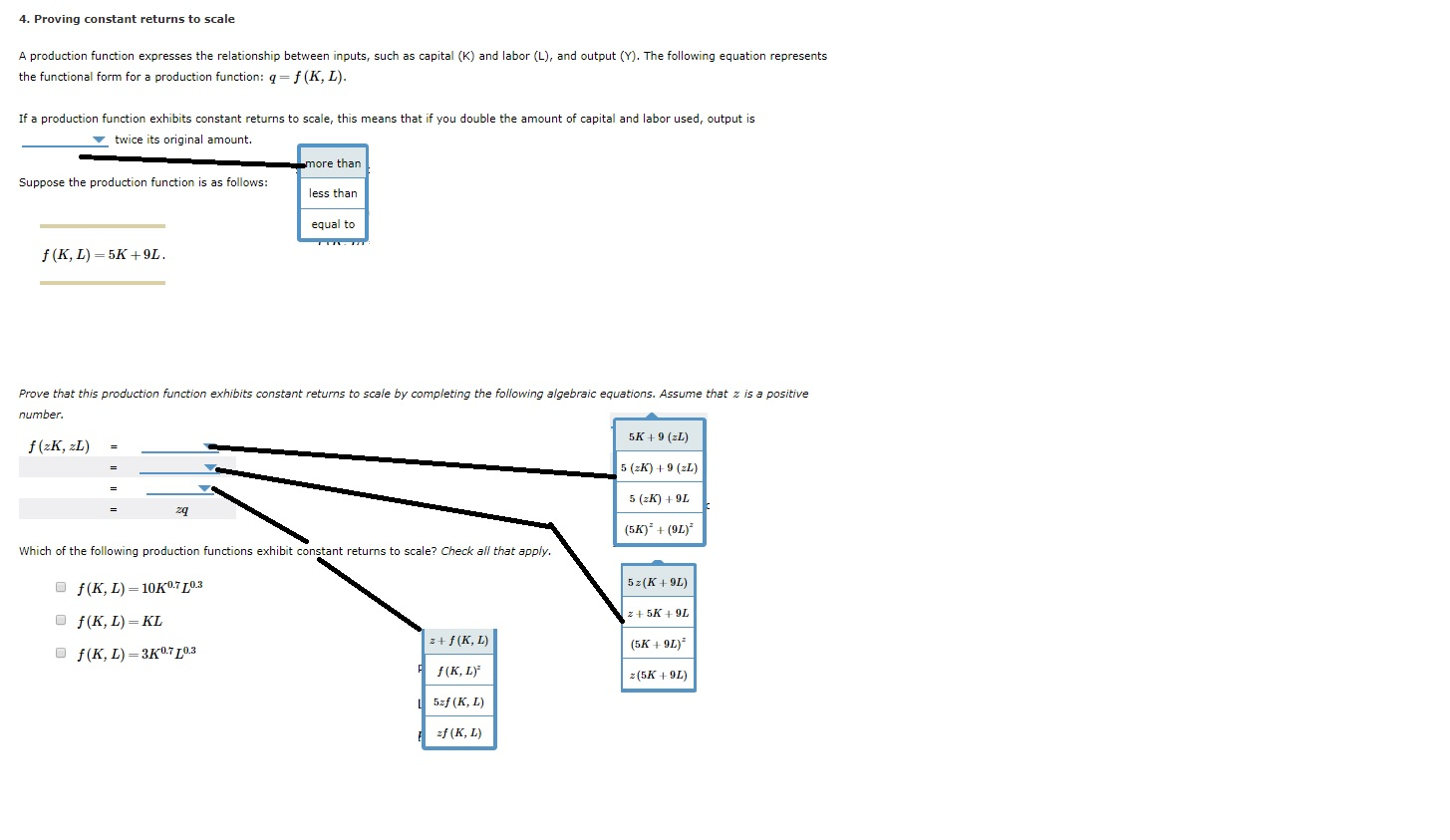4. Proving constant returns to scale A production function expresses the relationship between inputs, such as capital (K) and labor (L), and output (Y). The following equation represents the functional form for a production function: 9=f(K, L). If a production function exhibits constant returns to scale, this means that if you double the amount of capital and labor used, output is twice its original amount. more than Suppose the production function is as follows: less than equal to f( KL)=5K+9L Prove that this production function exhibits constant returns to scale by completing the following algebraic equations. Assume that z is a positive number. 5K+9 (2L) f (2K, 2L) = 5 (2K) +9 (2L) 5 (2K) +9L (5K) +(91) Which of the following production functions exhibit constant returns to scale? Check all that apply. f(KL)= 10K07/03 5z(K +9L) z+5K +9L f(K, L)=KL z+f(KL) (5K +9L) f(KL)- 307[0.3 f(KL) z (5K +9L) 5zf (KL) zf (KL)

#### Homework Answers

Answer #1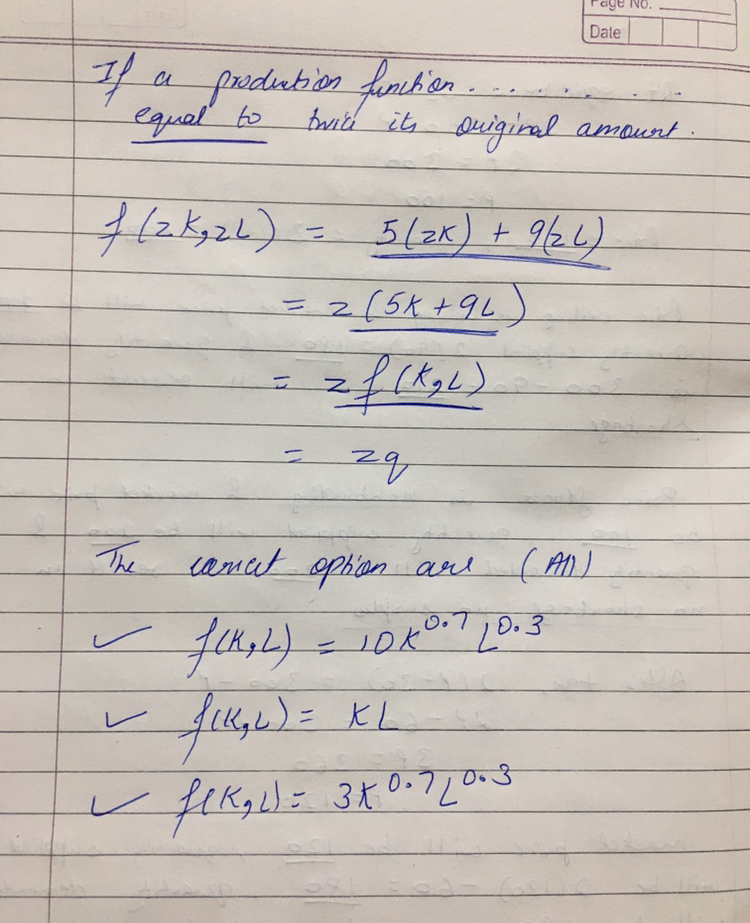Know the answer?
Your Answer:

#### Post as a guest

Your Name:

What's your source?

#### Earn Coin

Coins can be redeemed for fabulous gifts.

Not the answer you're looking for? Ask your own homework help question. Our experts will answer your question WITHIN MINUTES for Free.
Similar Homework Help Questions
• ### 1. Returns to scale. A production function has constant returns to scale with respect to inputs w...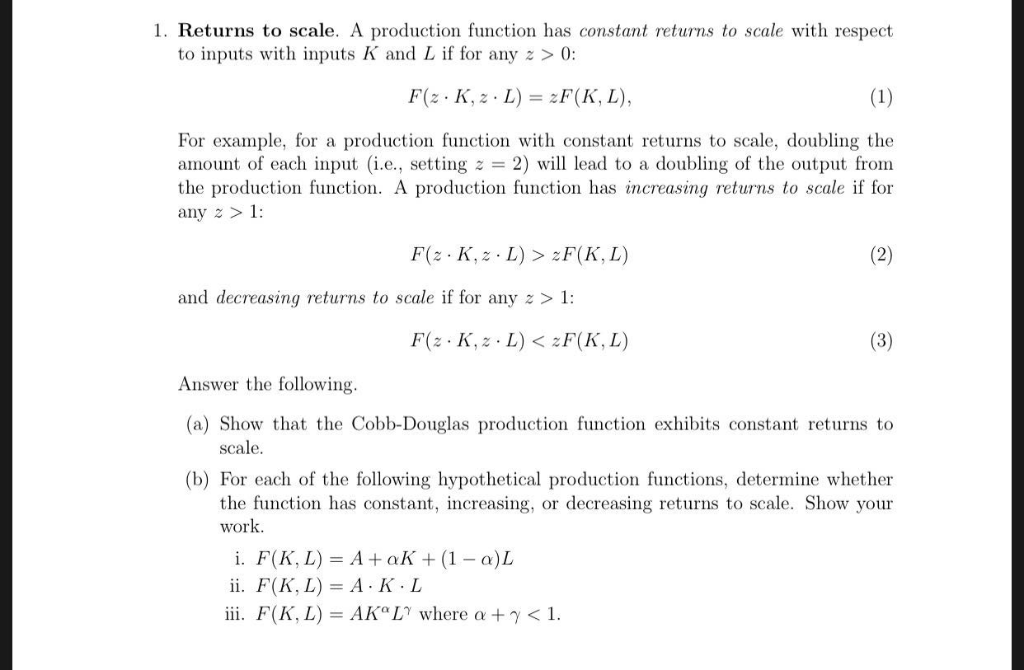1. Returns to scale. A production function has constant returns to scale with respect to inputs with inputs K and L if for any z >0: F(z . K, z、L) = zF(K, L), For example, for a production function with constant returns to scale, doubling the anount of each input (i.e., setting z = 2) will lead to a doubling of the output from the production function. A production function has increasing returns to scale if for any z and...

• ### 1. Returns to scale. A production function has constant returns to scale with respect to inputs...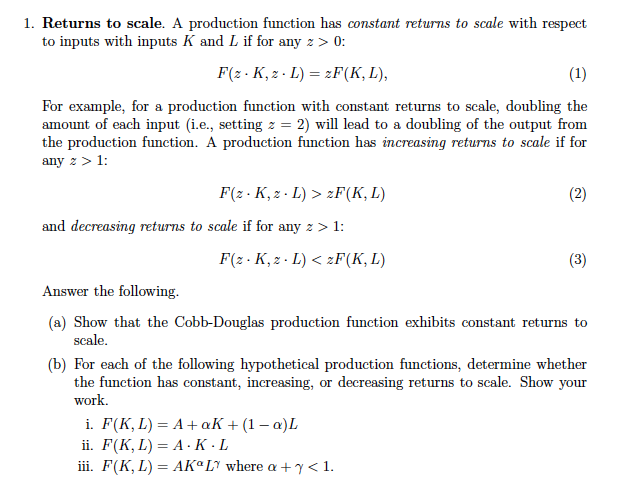1. Returns to scale. A production function has constant returns to scale with respect to inputs with inputs K and L if for any z 0: For example, for a production function with constant returns to scale, doubling the amount of each input (i.e., setting z = 2) will lead to a doubling of the output from returns to scale if for function has in any z > 1: and decreasing returns to scale if for any z>1 Answer the...

• ### 13) Does the production function of Table 7-1 show constant, increasing or decreasing returns to scale...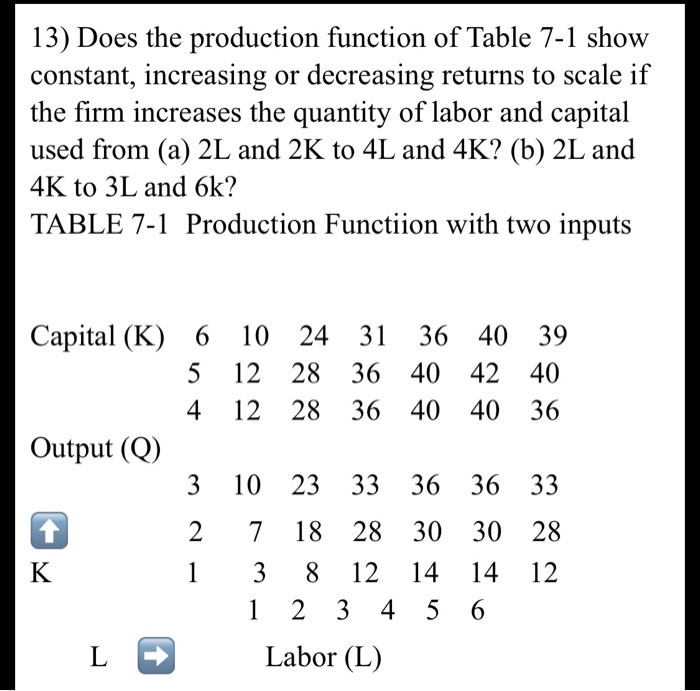13) Does the production function of Table 7-1 show constant, increasing or decreasing returns to scale if the firm increases the quantity of labor and capital used from (a) 2L and 2K to 4L and 4K? (b) 2L and 4K to 3L and 6k? TABLE 7-1 Production Functiion with two inputs Capital (K) 6 10 24 31 36 40 39 5 12 28 3640 42 40 4 12 28 36 40 40 36 Output (Q) 3 10 23 33 36...

• ### 1. Below are production functions that turn capital (K) and labor (L) into output. For each...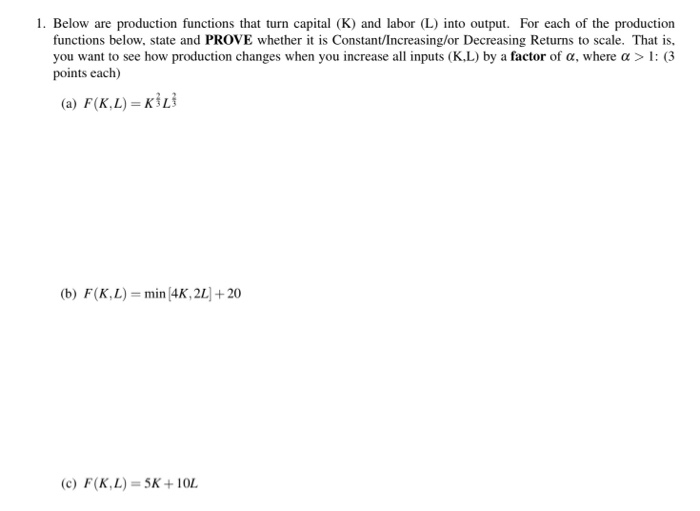1. Below are production functions that turn capital (K) and labor (L) into output. For each of the production functions below, state and PROVE whether it is Constant/Increasing/or Decreasing Returns to scale. That is, you want to see how production changes when you increase all inputs (KL) by a factor of a, where a > 1: (3 points each) (a) F(K.L) = (b) F(KL)= min (4K, 2L + 20 (c) F(K,L) = 5K+ 10L

• ### Q#02 Check whether the following production function exhibits (10 Marks) Constant Returns to Scale Increasing Returns...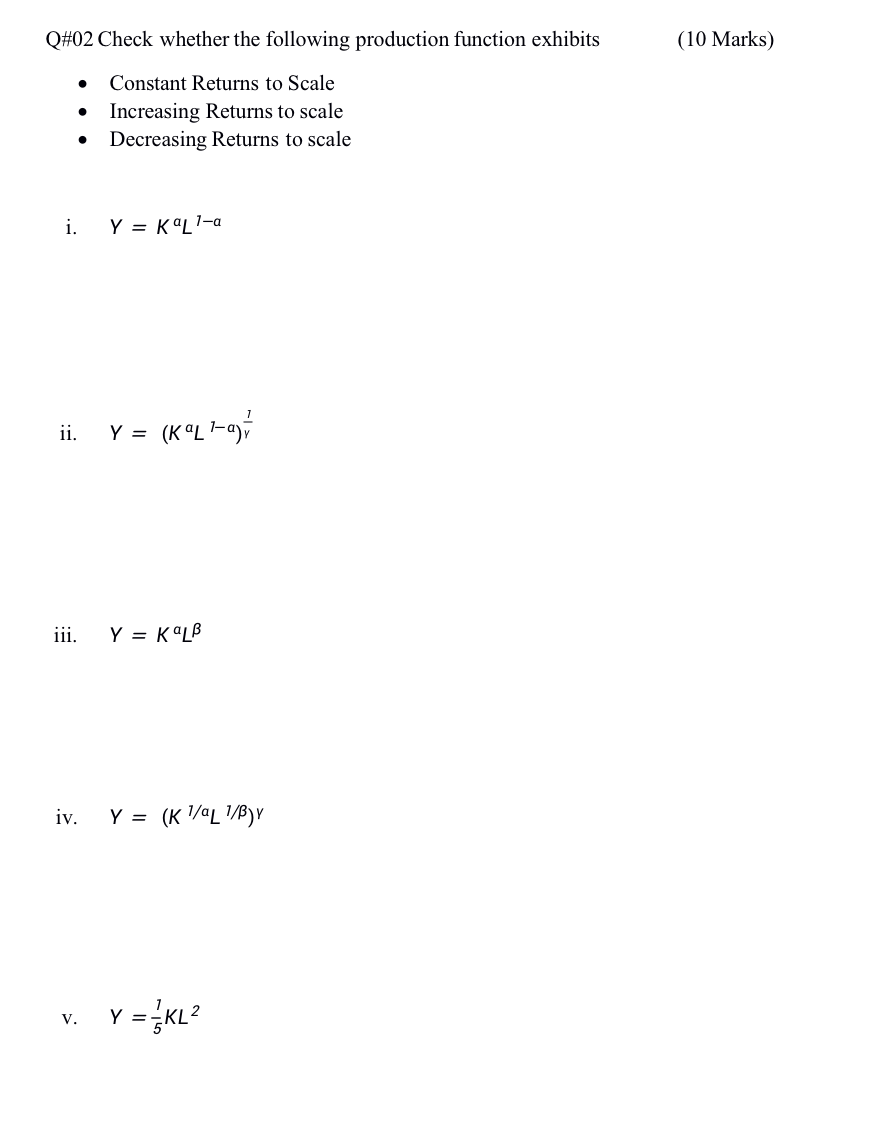Q#02 Check whether the following production function exhibits (10 Marks) Constant Returns to Scale Increasing Returns to scale Decreasing Returns to scale . i. Y = Kal1-a ii. Y = (KL-ay iii. Y = KOLB iv. Y = (K 1/4L 1/8), v. Y = KL

• ### A production function exhibits constant returns to scale if: Doubling all inputs delivers exactly twice the...

A production function exhibits constant returns to scale if: Doubling all inputs delivers exactly twice the output. Doubling all inputs delivers exactly more than twice the output. Doubling all inputs delivers exactly less than twice the output. none of the above The marginal product of capital (MPK) is: The additional unit of output that is produced when both labor and capital are increased by one unit. The additional output that is produced when there is technological improvement. The additional output...

• ### Which of the following production funcitons exhibits constant returns to​ scale? A. q​ = log(KL) B....

Which of the following production funcitons exhibits constant returns to​ scale? A. q​ = log(KL) B. q​ = K​ + L C. q​ = KL0.5 D. q​ = KL

• ### Does the production function of table 7-1 below show constant, increasing, or decreasing return to scale...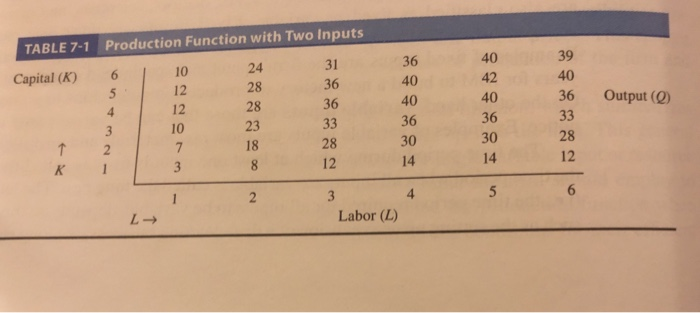Does the production function of table 7-1 below show constant, increasing, or decreasing return to scale if the firm increases the quantity of labor and capital used from (a) 2L and 2K to 4L and 4K? (b) 2L and 4K to 3L and 6K? TABLE 7-1 Production Function with Two Inputs 36 40 40 36 30 14 40 42 40 36 30 14 39 40 36 Output (0) 10 12 12 10 24 28 28 23 18 31 36 36...

• ### 10. Verify that If the production function exhibits constant returns to scale, the cost function may...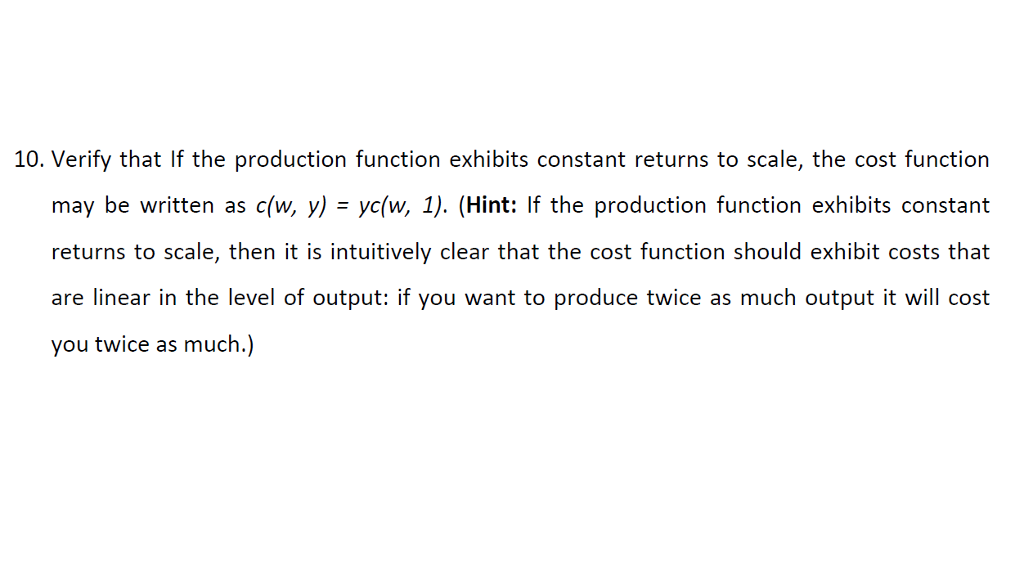10. Verify that If the production function exhibits constant returns to scale, the cost function may be written as c(w, y)-ye(w, 1). (Hint: If the production function exhibits constant returns to scale, then it is intuitively clear that the cost function should exhibit costs that are linear in the level of output: if you want to produce twice as much output it will cost you twice as much.)

• ### Homework 2 Question 4. Show that the Cobb-Douglas production function has constant returns to scale. Hint:...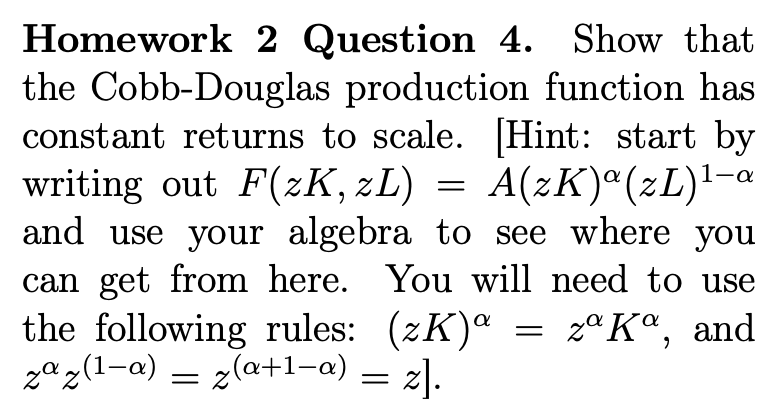Homework 2 Question 4. Show that the Cobb-Douglas production function has constant returns to scale. Hint: start by writing out F(2K, 2L)-A(2K)"CL)1-a and use your algebra to see where you can get from here. You will need to use the following rules: (2K)α-ΖαΚ®, and

Free Homework App

Scan Your Homework
to Get Instant Free Answers
Need Online Homework Help?

Get Answers For Free
Most questions answered within 3 hours.